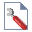The Rocscience International Conference 2021 Proceedings are now available. Read Now

Probabilistic Analysis Overview

Probabilistic analysis can be carried out in RS2 (version 9) using the Point Estimate, Monte Carlo or Latin Hypercube methods. The general procedure is as follows.

Project Settings

In the Project Settingsdialog, select the Probabilistic Analysis option and choose the Sampling Method (Point Estimate, Monte Carlo, Latin Hypercube) and Number of Samples if applicable.

Random Variables

In order to carry out a Probabilistic analysis, you must define one or more input parameters as random variables, by choosing the variables and entering the required statistical parameters (e.g. distribution, mean, standard deviation). Random variables are defined using the options in the Statistics menu. You may define material properties, joint properties and field stress as random variables.

Material Property Statistics

Joint Property Statistics

Field Stress Statistics

Future versions of RS2 may allow additional random variables. See the Random Variables topic for more information.

NOTE: any input parameter which is NOT defined as a random variable, is assumed to be exactly known (by the Mean value), and will not be involved in the statistical sampling process.

Compute

Select Compute and the finite element analysis will be computed for the Number of Samples specified (Monte Carlo or Latin Hypercube methods), or for each possible combination of random variable point estimates (Point Estimate Method).

Probabilistic Analysis Results

Select Interpret and you will be able to view contours of statistical results and generate error plots of query data. The following statistical results are available:

• Contours of the mean, standard deviation and coefficient of variation for all output variables (e.g. stress, displacement)
• Error plots for material queries, showing the statistical range of output values at a user-defined query location (e.g. statistical variation of stress or displacement along a tunnel boundary)
• Display of yield zone probability
• Probability of failure (SSR slope stability analysis)